# ACT Math Practice Test 5

Difficulty Level – 3: Medium / Difficult

Directions: Solve each problem and then click on the correct answer. You are permitted to use a calculator on this test.

Congratulations - you have completed . You scored %%SCORE%% out of %%TOTAL%%. Your performance has been rated as %%RATING%%
 Question 1
A number, x, is decreased by 40% then increased by 25%. What is the final result in terms of x?

 A 0.55x B 0.575x C 0.65x D 0.7x E 0.75x
Question 1 Explanation:
The correct answer is (E). To solve this, set x = 100 and perform the operations. One hundred is a great number to choose here because 1% of 100 is 1.

100 − 0.4 * 100 = 60

60 + 0.25 * 60 = 75

Because x = 100 was our initial value, we want to choose the answer choice that gives us 75 when we substitute 100 for x, 0.75 * 100 = 75, so answer choice (E) is correct.
 Question 2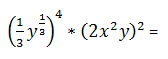A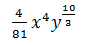B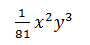C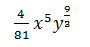D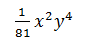E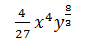Question 2 Explanation:
The correct answer is (A). Evaluate the expression.
13 to the 4th power is 181 and y13 raised to the 4th power is 43 so the first expression is y43 divided by 81.

The second expression evaluates to 4 * x4 * y2. Our coefficient becomes 481 and our x is to the 4th power and when multiplying y43 and y2, we add the exponents to get y103.
 Question 3
The expression (x + y) [z − (x − y)] is equivalent to which of the following?

 A xz − x2 + 2xy + y2 B 2xz + x2 − yz + y2 C xz − x2 + yz + y2 D xz + x2 − yz − y2 E xyz + y2 − xy + yz + y2
Question 3 Explanation:
The correct answer is (C). Recall order of operations and the distributive property to answer this question. The second bracket simplifies to zx + y and to this we multiply (x + y). Begin by distributing the x through (zx + y) and to this add the distribution of y through (zx + y) to get answer choice (C).
 Question 4
There are 32 marbles in a jar: 14 blue, 10 red, 5 green, and 3 yellow. Sally pulls one marble, randomly, from the jar. Without replacing the marble, she pulls another marble. What is the probability that both marbles will be red?

 A 40⁄426 B 41⁄456 C 43⁄476 D 44⁄476 E 45⁄496
Question 4 Explanation:
The correct answer is (E). The probability of selecting a red marble on the first draw is 1032 because there are 10 red marbles and 32 total marbles. After removing the first red marble there are now 9 red marbles and 31 total marbles left so 931 chance of selecting the second red marble. To find the probability of both events occurring, we multiply the probabilities to get 9 * 1032 * 31 which reduces to 45496.
 Question 5
If x + y = 7 and xy = 3, what is x2 − y2 = ?

 A 4 B 21 C 25 D 36 E 40
Question 5 Explanation:
The correct answer is (B). We can either use the system of equations to solve for x and y individually to find that x = 5 and y = 2 which gives 25 − 4 = 21, or we can see that if we multiply (x + y) and (xy) we will be left with x2y2, 7 * 3 = 21.
 Question 6
The circumference of a large wedding cake is 60 inches. If the cake is divided evenly into 12 slices, what is the length of the arc, in inches, made by 5 combined slices?

 A 12 B 13 C 15 D 20 E 25
Question 6 Explanation:
The correct answer (E). Recall that the circumference is the distance around a circle. If the cake is divided into 12 equal slices, each arc length will be 5. Summing 5 of these slices will give 25.
 Question 7
A rectangle measures 65 inches by 77 inches. What is the length of the diagonal of the rectangle (rounded to the nearest hundredth)?

 A 100.77 B 110.77 C 125.07 D 134.67 E 137.77
Question 7 Explanation:
The correct answer is (A). Use the Pythagorean Theorem to solve this problem. Recall that the sum of the side lengths squared equals the hypotenuse squared, so 652 + 772 = diagonal2. Take the square root of both sides to find the length and round to the nearest hundredth.
 Question 8
If ƒ(x) = x2 + 2x + 2, what is ƒ(x + h)?

 A 2x2 + 4hx + h2 − 2x − 2h B x + h2 + 2xh + 2 + h C x2 + 2x + 2xh + 2h + h2 + 2 D x2 + 4xh + 4h2 + 2x E 2x2 + 2xh + 2h2 + 2x + 2
Question 8 Explanation:
The correct answer is (C). To solve this problem we will substitute (x + h) for every x in our function ƒ(x):
ƒ(x +h) = (x +h)2 + 2(x +h) + 2.

Evaluate the expression using the distributive property to arrive at:
x2 + 2x + 2xh + 2h + h2 + 2.
 Question 9In the figure above, lines d and f are parallel and the angle measures are as given. What is the value of x?

 A 35 B 60 C 85 D 100 E 120
Question 9 Explanation:
The correct answer is (C). Since vertical angles are congruent, the angle vertical to the 35-degree angle also has a measure of 35 degrees. The supplement of the 120-degree angle has a measure of 60 degrees, so we then have a triangle with angles measuring 35, 60, and x degrees, as shown in the figure provided. Since the angles of a triangle add to 180 degrees:
35 + 60 + x = 180
x = 180 − 35 − 60
x = 85 degrees
 Question 10
Which of the following represents the equation of the line that passes through the point (2, 3) with a slope of −⅓?

 A y = 2x + 4 B y = 4x + 2 C y = −⅓x + 11⁄3 D y = −3x + 11⁄3 E y = −2⁄3x + 11⁄3
Question 10 Explanation:
The correct answer is (C). Recall that when provided with a point and the slope of a line, we can use point-slope formula to write an equation for the line. The point slope formula is yy1 = m(xx1) where (x1, y1) is the point provided and m is the slope. Plug in the point and slope provided and solve for y:
y − 3 = −⅓ (x − 2)
y = −⅓x + 113
Once you are finished, click the button below. Any items you have not completed will be marked incorrect.
There are 10 questions to complete.
 ← List →